# RS Aggarwal Solutions for Class 8 Chapter 7 - Factorisation Exercise 7D

Students can refer and download RS Aggarwal Solutions for Class 8 Maths Chapter 7- Exercise 7D, Factorisation from the links provided below. Our experts have solved the RS Aggarwal Solutions to ensure that the students are thorough with their basic concepts by practising the solutions. In Exercise 7D of RS Aggarwal Class 8 Maths, we shall study one of the methods i.e. Factorisation of quadratic trinomials.

## Download PDF of RS Aggarwal Solutions for Class 8 Maths Chapter 7- Exercise 7D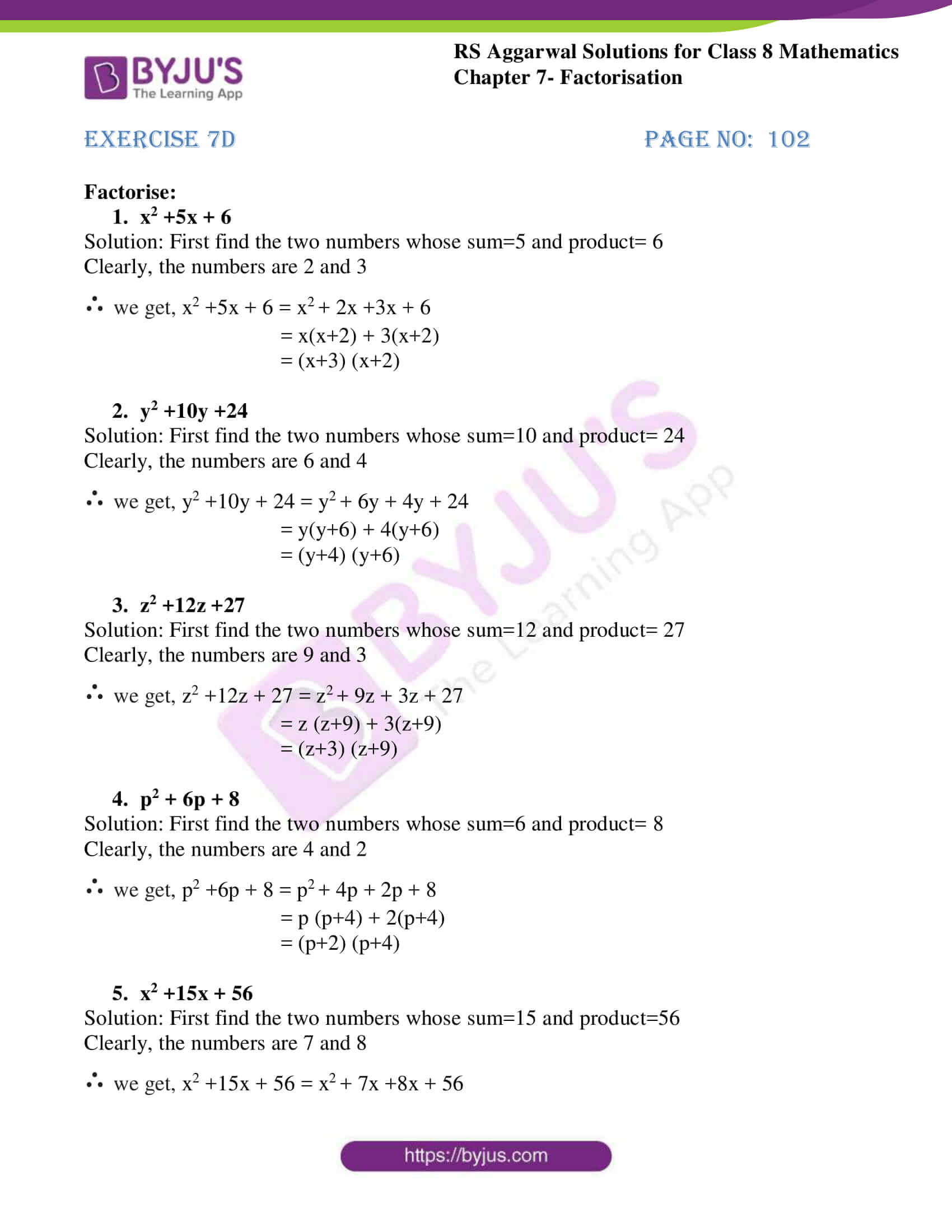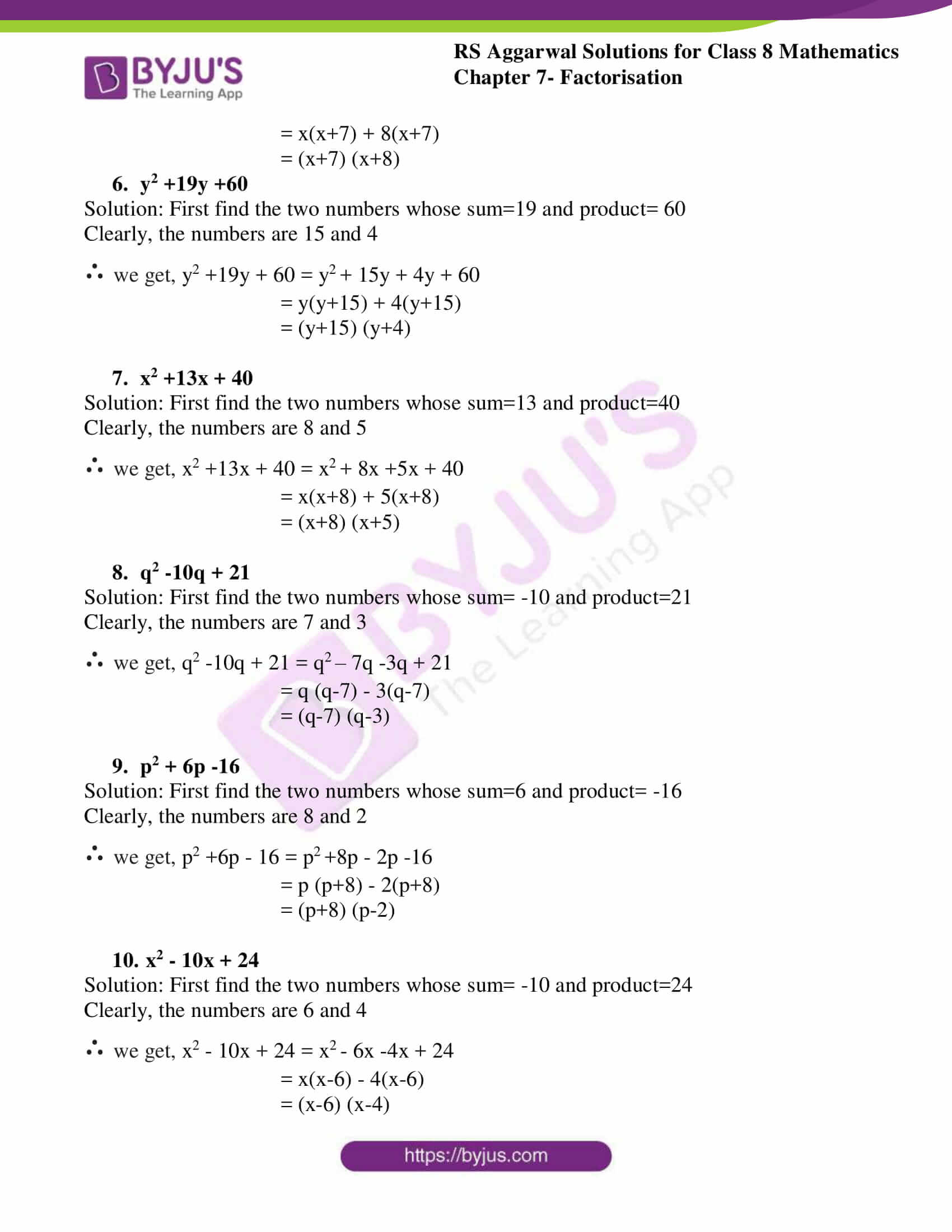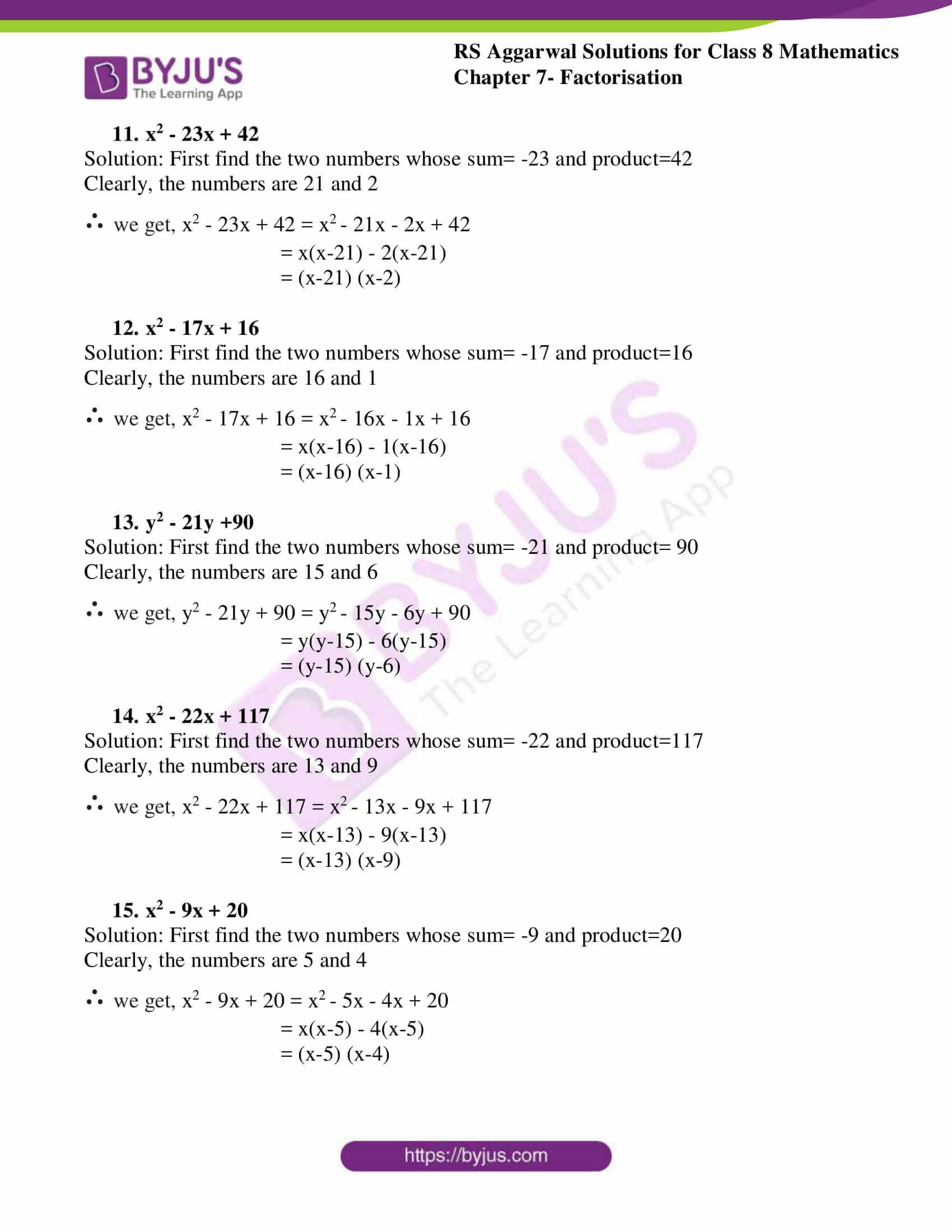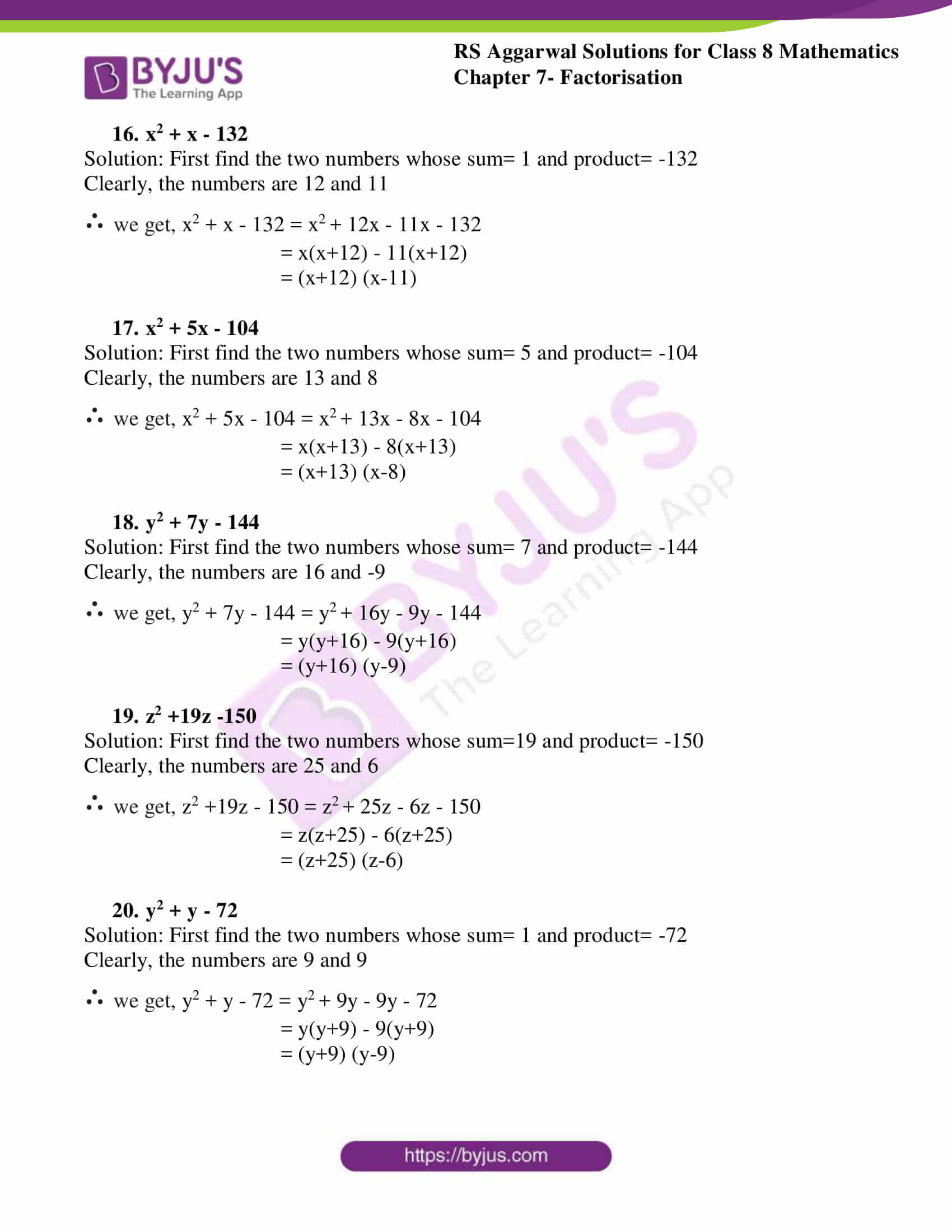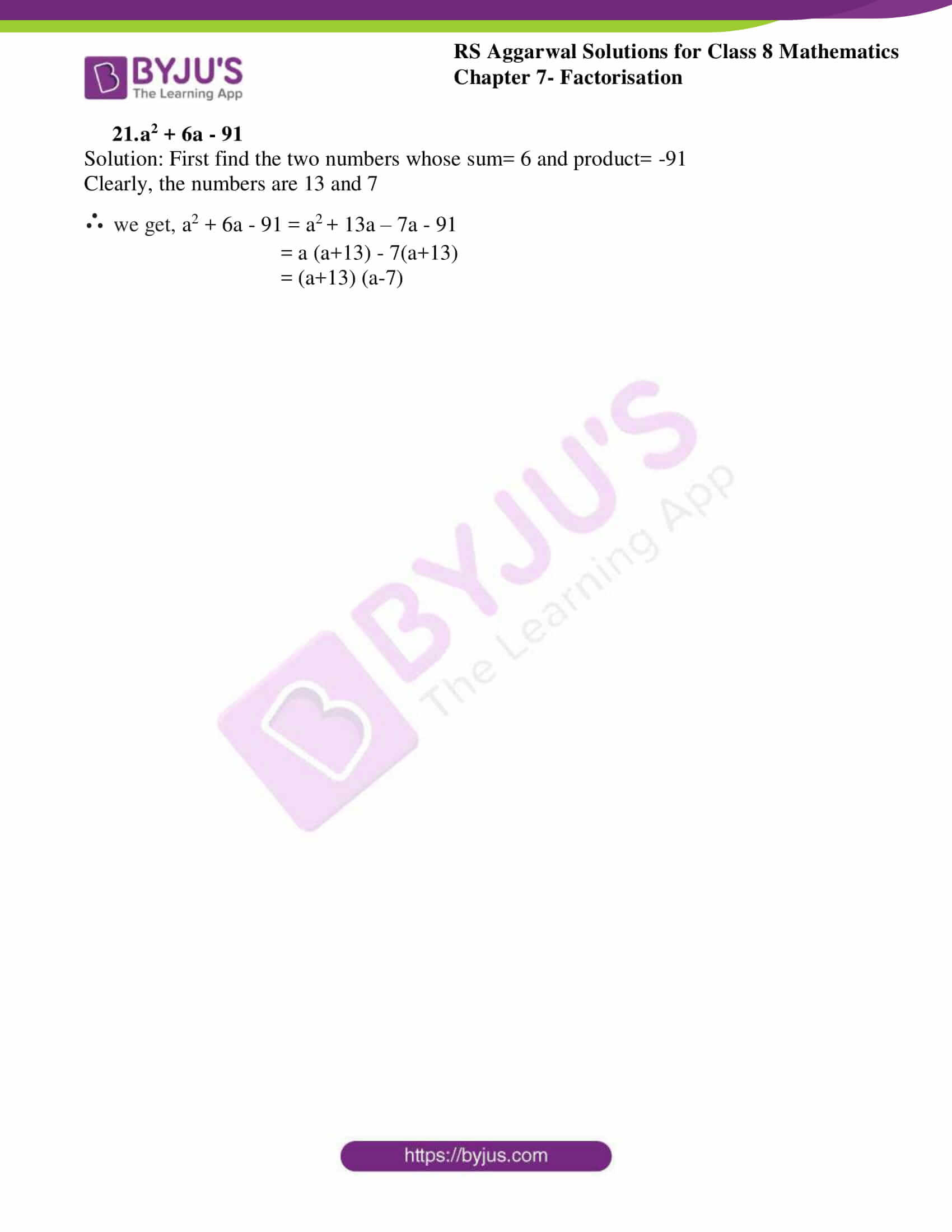### Access Answers to RS Aggarwal Solutions for Class 8 Maths Chapter 7- Factorisation Exercise 7D

Factorise:

1. x2 +5x + 6

Solution: First find the two numbers whose sum=5 and product= 6

Clearly, the numbers are 2 and 3

∴ we get, x2 +5x + 6 = x2 + 2x +3x + 6

= x(x+2) + 3(x+2)

= (x+3) (x+2)

2. y2 +10y +24

Solution: First find the two numbers whose sum=10 and product= 24

Clearly, the numbers are 6 and 4

∴ we get, y2 +10y + 24 = y2 + 6y + 4y + 24

= y(y+6) + 4(y+6)

= (y+4) (y+6)

3. z2 +12z +27

Solution: First find the two numbers whose sum=12 and product= 27

Clearly, the numbers are 9 and 3

∴ we get, z2 +12z + 27 = z2 + 9z + 3z + 27

= z (z+9) + 3(z+9)

= (z+3) (z+9)

4. p2 + 6p + 8

Solution: First find the two numbers whose sum=6 and product= 8

Clearly, the numbers are 4 and 2

∴ we get, p2 +6p + 8 = p2 + 4p + 2p + 8

= p (p+4) + 2(p+4)

= (p+2) (p+4)

5. x2 +15x + 56

Solution: First find the two numbers whose sum=15 and product=56

Clearly, the numbers are 7 and 8

∴ we get, x2 +15x + 56 = x2 + 7x +8x + 56

= x(x+7) + 8(x+7)

= (x+7) (x+8)

6. y2 +19y +60

Solution: First find the two numbers whose sum=19 and product= 60

Clearly, the numbers are 15 and 4

∴ we get, y2 +19y + 60 = y2 + 15y + 4y + 60

= y(y+15) + 4(y+15)

= (y+15) (y+4)

7. x2 +13x + 40

Solution: First find the two numbers whose sum=13 and product=40

Clearly, the numbers are 8 and 5

∴ we get, x2 +13x + 40 = x2 + 8x +5x + 40

= x(x+8) + 5(x+8)

= (x+8) (x+5)

8. q2 -10q + 21

Solution: First find the two numbers whose sum= -10 and product=21

Clearly, the numbers are 7 and 3

∴ we get, q2 -10q + 21 = q2 – 7q -3q + 21

= q (q-7) – 3(q-7)

= (q-7) (q-3)

9. p2 + 6p -16

Solution: First find the two numbers whose sum=6 and product= -16

Clearly, the numbers are 8 and 2

∴ we get, p2 +6p – 16 = p2 +8p – 2p -16

= p (p+8) – 2(p+8)

= (p+8) (p-2)

10. x2 – 10x + 24

Solution: First find the two numbers whose sum= -10 and product=24

Clearly, the numbers are 6 and 4

∴ we get, x2 – 10x + 24 = x2 – 6x -4x + 24

= x(x-6) – 4(x-6)

= (x-6) (x-4)

11. x2 – 23x + 42

Solution: First find the two numbers whose sum= -23 and product=42

Clearly, the numbers are 21 and 2

∴ we get, x2 – 23x + 42 = x2 – 21x – 2x + 42

= x(x-21) – 2(x-21)

= (x-21) (x-2)

12. x2 – 17x + 16

Solution: First find the two numbers whose sum= -17 and product=16

Clearly, the numbers are 16 and 1

∴ we get, x2 – 17x + 16 = x2 – 16x – 1x + 16

= x(x-16) – 1(x-16)

= (x-16) (x-1)

13. y2 – 21y +90

Solution: First find the two numbers whose sum= -21 and product= 90

Clearly, the numbers are 15 and 6

∴ we get, y2 – 21y + 90 = y2 – 15y – 6y + 90

= y(y-15) – 6(y-15)

= (y-15) (y-6)

14. x2 – 22x + 117

Solution: First find the two numbers whose sum= -22 and product=117

Clearly, the numbers are 13 and 9

∴ we get, x2 – 22x + 117 = x2 – 13x – 9x + 117

= x(x-13) – 9(x-13)

= (x-13) (x-9)

15. x2 – 9x + 20

Solution: First find the two numbers whose sum= -9 and product=20

Clearly, the numbers are 5 and 4

∴ we get, x2 – 9x + 20 = x2 – 5x – 4x + 20

= x(x-5) – 4(x-5)

= (x-5) (x-4)

16. x2 + x – 132

Solution: First find the two numbers whose sum= 1 and product= -132

Clearly, the numbers are 12 and 11

∴ we get, x2 + x – 132 = x2 + 12x – 11x – 132

= x(x+12) – 11(x+12)

= (x+12) (x-11)

17. x2 + 5x – 104

Solution: First find the two numbers whose sum= 5 and product= -104

Clearly, the numbers are 13 and 8

∴ we get, x2 + 5x – 104 = x2 + 13x – 8x – 104

= x(x+13) – 8(x+13)

= (x+13) (x-8)

18. y2 + 7y – 144

Solution: First find the two numbers whose sum= 7 and product= -144

Clearly, the numbers are 16 and -9

∴ we get, y2 + 7y – 144 = y2 + 16y – 9y – 144

= y(y+16) – 9(y+16)

= (y+16) (y-9)

19. z2 +19z -150

Solution: First find the two numbers whose sum=19 and product= -150

Clearly, the numbers are 25 and 6

∴ we get, z2 +19z – 150 = z2 + 25z – 6z – 150

= z(z+25) – 6(z+25)

= (z+25) (z-6)

20. y2 + y – 72

Solution: First find the two numbers whose sum= 1 and product= -72

Clearly, the numbers are 9 and 9

∴ we get, y2 + y – 72 = y2 + 9y – 9y – 72

= y(y+9) – 9(y+9)

= (y+9) (y-9)

21. a2 + 6a – 91

Solution: First find the two numbers whose sum= 6 and product= -91

Clearly, the numbers are 13 and 7

∴ we get, a2 + 6a – 91 = a2 + 13a – 7a – 91

= a (a+13) – 7(a+13)

= (a+13) (a-7)

## RS Aggarwal Solutions for Class 8 Maths Chapter 7- Exercise 7D

Exercise 7D of RS Aggarwal Class 8, Factorisation. This exercise mainly deals with Factorising a quadratic trinomial. The RS Aggarwal Solutions can help the students practice diligently while learning the fundamentals as it provides all the answers to the questions from the RS Aggarwal textbook. Students are suggested to practice the problems on a regular basis which will help them excel in their exams and increase their overall percentage. Practising as many times as possible helps in accelerating time management abilities and also pushes up the confidence level to achieve high marks.